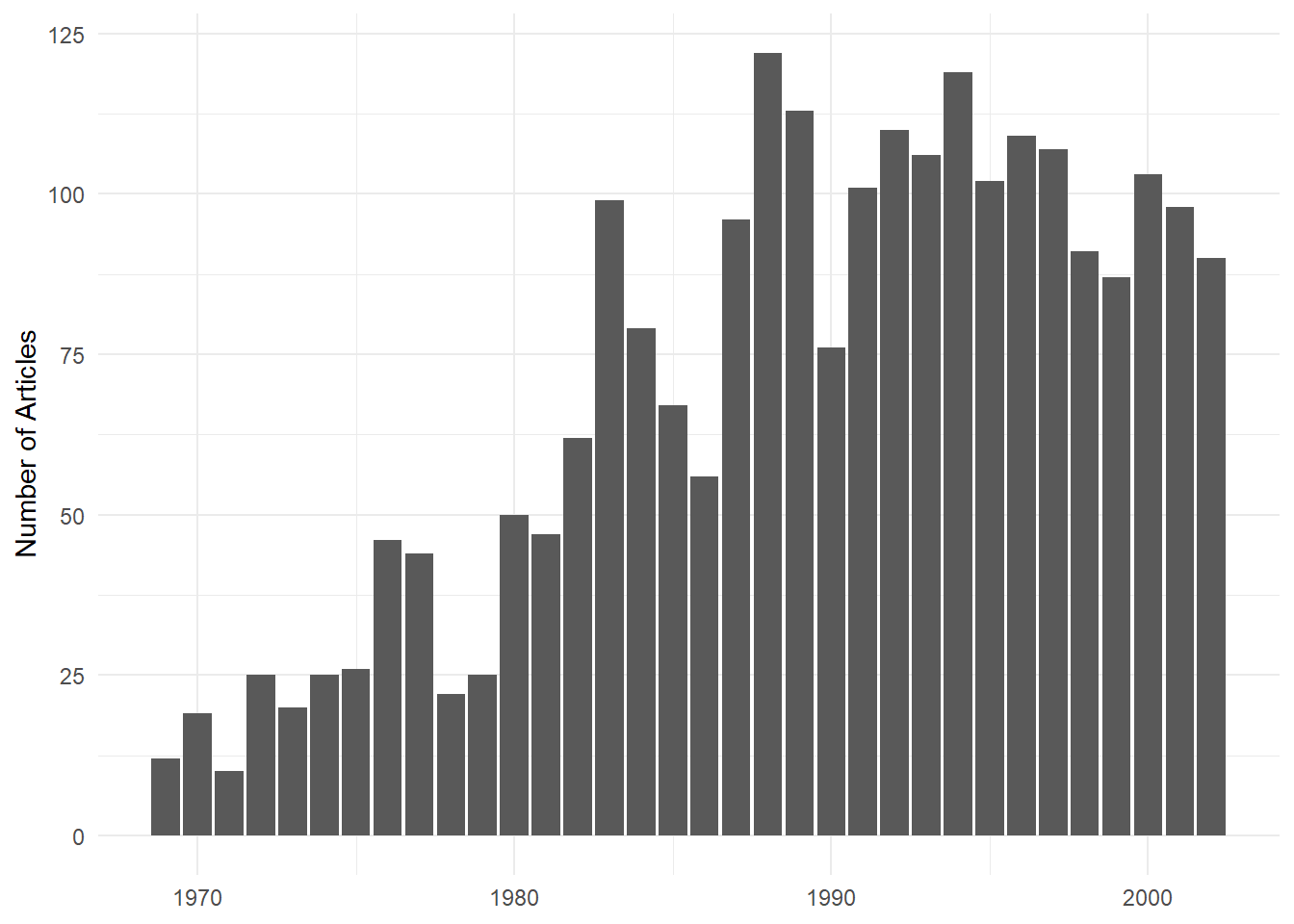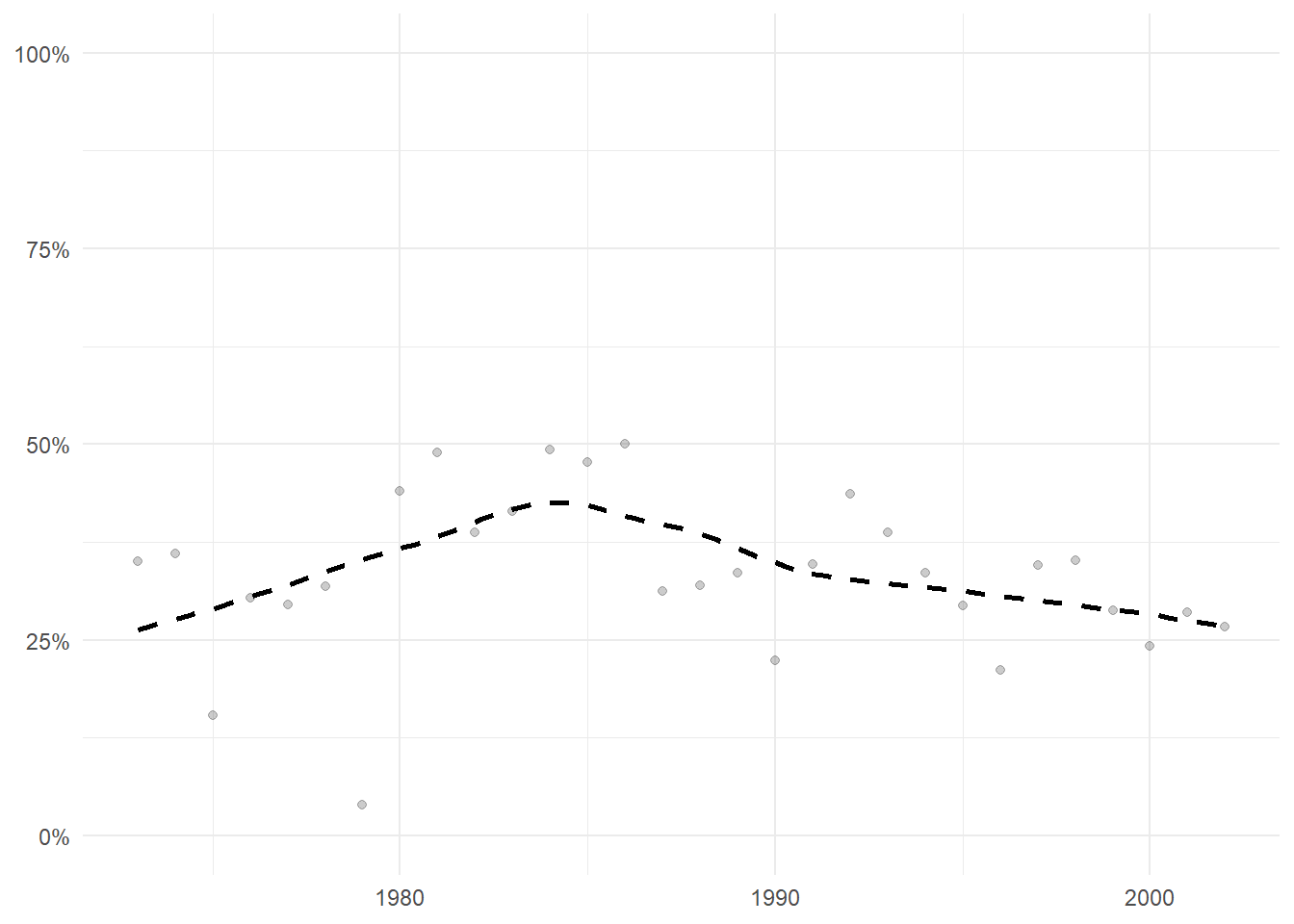# 1 Introduction

In the section EER Bibliographic Information of this appendix, we compiled bibliographic information related to all articles published in the European Economic Review (EER). The goal of this section is to provide general information about the journal, to contextualize the following network analysis. Users can explore information about the most cited references, the most productive authors in the journal, or the type of collaboration between US and European economists in the journal.

In the section Dynamics Networks, we propose a network analysis of all macro articles, identified by JEL codes, published in the top five economics journals and the EER. In this section, we explore the structure of macroeconomics publications in these journals by identifying clusters of articles that tackle similar subjects using their bibliography. From these clusters, we identify clusters that are more European-oriented (research published in the EER and/or written by European authors) from clusters that are more US-oriented.

Finally, in section A Look at Dynamic Communities users can explore information about the individual clusters identified in the previous section. For example, we produced a variety of tables to explore the most cited references of the cluster or its most distinctive words/institutions compared to the overall corpus. In addition, users can also find some intra-clusters information about how the cluster evolved overtime, or the differences in the clusters between European/US authors and/or venues of publications.

# 2 EER Bibliographic Information

In this section we briefly explore some of the information compiled about the EER, its relationship to macro and the importance of the EER in the overall landscape of economics.

## 2.1 Distribution of EER Articles

``````ggplot(Corpus_all_EER, aes(as.numeric(Annee_Bibliographique))) +
geom_bar() +
labs(x = NULL,
y = "Number of Articles") +
theme_minimal() ``````## 2.2 Share Macro

For each year, we calculated the share of articles published in the EER that matched macroeconomics JEL codes to get get an idea of the changing importance of macroeconomics in the journal:

``````mean_jel <- Corpus_all_EER[,mean(jel_id),Annee_Bibliographique]

ggplot(mean_jel[V1!=0 & Annee_Bibliographique!="2016"], aes(x=as.numeric(Annee_Bibliographique), y=V1)) +
geom_smooth(method="auto", se=FALSE, fullrange=FALSE, level=0.95, span = 0.75,color="black", linetype = "dashed")+
geom_point(alpha=0.2)+
theme(axis.text.x = element_text(angle = 90, vjust = 0.5)) +
labs(x = NULL,
y = NULL) +
scale_y_continuous(labels = scales::label_percent()) +
scale_x_continuous() +
coord_cartesian(ylim = c(0,1)) +
theme_minimal() ````````````  # theme(plot.background = element_rect(fill = 'white', colour = NA)) +
ggsave(here(eer_data,"pictures","Graphs","mean_jel.png"), width=30, height=20, units = "cm", scaling = 1.7)

# ragg::agg_png(here(eer_data,"pictures","Graphs","ragg.png"), width = 30, height = 20, units = "cm", res = 300, scaling = 2)``````

## 2.3 Collaborations between Europeans and US in the EER

The following graph informs us about the collaborations between US and European authors in the EER: